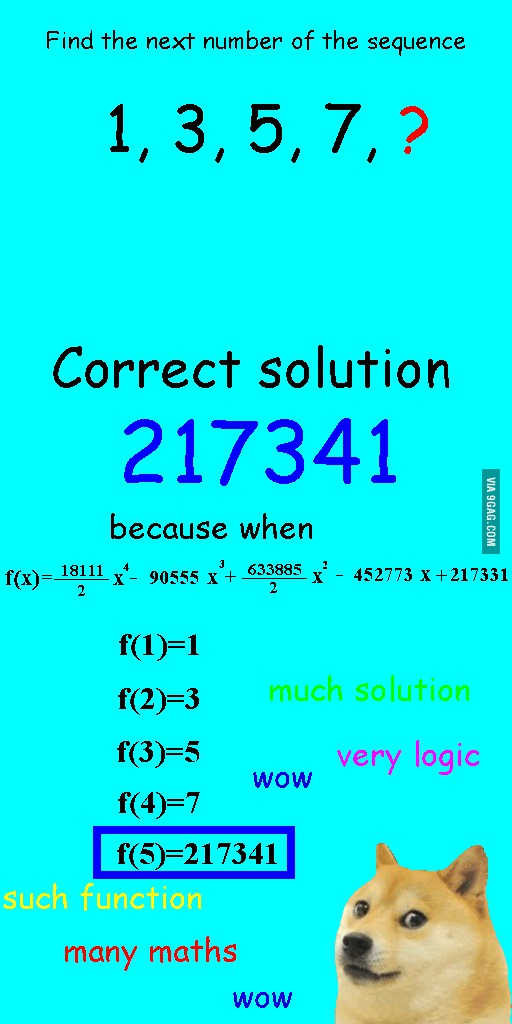# Discrediting Questions about the Next Number in a Series with the Lagrange PolynomialFor quite some time I have been annoyed by the kind of IQ test questions where you are given a series of numbers, quite often without any explanation and are expected to provide the following number in the series. These are the type of questions I fail most often on IQ tests and maybe this is the reason I hate them so much. I can accept that I am stupid but I cannot accept people claiming that these are Math problems. This is not simply not Math. This is anti-Math.

In Math you are never given a series of numbers that you have to recognize. You are given something that defines the series and need to find certain member. The reason this is the case is that a series can be defined simply by listing its members. For example you can answer 1, 3, 5, 7, ? with 3.14 and this is perfectly valid mathematical solution because you simply considered the series defined by members 1, 3, 5, 7, 3.14.

Recently even stronger argument in the form of a Doge meme made the rounds on the Internet.It turns out for whatever series of non-repeating numbers you can find a polynomial of minimal degree with a formula. After some digging I found out that this is called Lagrange Polynomial. I even vaguely remembered that it was part of my university Math course. I decided to write a program to produce the polynomial for whatever numbers the user puts in so I can prove that all these series questions make no sense at all. What is the next number you ask? What do you want it to be? Tell me and I will give you the function.

To do this I needed to use what is known as symbolic computation. Symbolic computation is programming where the results are not numbers but expressions with variables. For example (a^2 + a + 3) + (-a^2 + 2a + 2) will result in 3*a + 5. If you want to do symbolic computation you either use a language for symbolic programming like Wolfram Language or a symbolic computation library for your language of choice.

As it happened a couple of days ago someone posted a link to his .NET symbolic computation library called Symbolism on Reddit. The example seemed pretty clear so I decided to try building the Lagrange polynomial thing with it. I was surprised that half an hour later I had a working program and most of the time was spent on understanding the Lagrange polynomial formulas on Wikipedia. Coding them was pretty straight forward and I got it right the first time minus some difficulties with the output. You can see my code here.

The interesting part is the code that calculates each basis polynomial of the Lagrange interpolation polynomial. Each basis polynomial is computed as the product of (X-Xm)/(Xj-Xm) where j is the index of the term and m goes from 0 to the number of pairs in the series. For better explanations and well-formatted formulas please check the Wikipedia article. With Symbolism it is straight-forward:

static MathObject GenerateBasisPolynomial(Symbol x, double[] xs, int index)
{
MathObject member = 1;
for (int i = 0; i < xs.Length; i++)
{
if (i != index)
{
member *= (x - xs[i]) / (xs[index] - xs[i]);
}
}

return member;
}

Then all that was left was to sum them:

var x = new Symbol("x");
var members = new MathObject[ys.Length];

for (int i = 0; i < ys.Length; i++)
{
members[i] = ys[i] * GenerateBasisPolynomial(x, xs, i);
}

var sum = new Sum(members);

It took me some time to find the extension method that expands the parenthesis:
return sum.AlgebraicExpand();

The result for

x0 = 1, y0 = 1, x1 = 2, y1 = 4, x3 = 3, y3 = 9
is
1 * x ^ 2

Now 1* is not needed but I can live with that. If we try the Doge meme example

x0 = 1, y0 = 1, x1 = 2, y1 = 3, x2 = 3, y2 = 5, x3 = 4, y3 = 7, x4 = 5, y4 = 217341
we get
217331 + -452773 * x + 316942.5 * x ^ 2 + -90555 * x ^ 3 + 9055.5 * x ^ 4.

This is exactly the Doge meme values except that the fractions are decimal, negative quotients are prefixed with a minus and added instead of subtracted and for some reason the higher power terms are at the end instead of the front. I guess it works.

Now we can all use this great software to prove that the questions about guessing what series the author was thinking about are not mathematical at all. If someone explains how to do this in Wolfram Alpha this article will be even more useless than it currently is.
Tags:   english programming
Posted by:   Stilgar
17:34 09.07.2015

# Posted by   Rhaegar (Unregistered)   on   12:30 10.07.2015

I'm shedding tears of joy to see this. :)

# Posted by   Guest (Unregistered)   on   01:39 22.05.2021

A few years late, but if you type "find polynomial with points (1,1) (2,3) (3,5) (4,7) (5,217341)" WolframAlpha will interpolate that for you: https://www.wolframalpha.com/input/?i=find+polynomial+with+points+%281%2C1%29+%282%2C3%29+%283%2C5%29+%284%2C7%29+%285%2C217341%29 . And of course, you can change, remove and add any points.

# Posted by   Guest (Unregistered)   on   01:44 22.05.2021

I do think it is a sort of mathematical question, even if worded poorly. Very often this question really wants to ask "what is the lowest degree polynomial that interpolates this given points". The problem is not with the way of solving it, but with the wrong question that is being asked.

# Posted by   Stilgar   on   12:11 30.05.2021

Well, the question in the meme above does not ask for the lowest degree it asks for the next number in an arithmetic progression.

792

Post as: